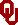# Galileo's Later Years

## The Law of Falling Bodies

Galileo’s Two New Sciences represents the culmination of a long line of mathematical investigations of motion, extending over 300 years up to Galileo’s unification of mathematics and physics. For example, in the 14th century Niccole Oresme produced a famous study of motion. Oresme’s Mean Speed Theorem bears a distinct resemblence to Galileo’s law of free fall, and was widely known. Indeed, the work of Oresme and other 14th century logicians provides a basis for Galileo’s boast that it is possible to deduce the law of free fall without recourse to observation.

Consider a graph of speed versus time (Figure 1) . If the speed increases by one unit for each unit of time; that is, if the speed increases by a constant amount per unit of time, then one is dealing with uniform acceleration (Figure 2) .

Since distance equals speed multiplied by time, the distance traveled in the first unit of time will be 1 unit of distance, or 1 triangle (Figure 3) . After the second unit of time, the distance will be 4 units, or 4 triangles (Figure 4) . After three units of time, the distance traveled will be 9 triangles; 16 triangles at time 4 (Figure 5) ; 25 triangles at time 5 (Figure 6) ; and a distance of 36 triangles will be traveled after 6 units of time (Figure 7) .

Another way of looking at this is to say that the distances traveled by a falling body increase as the square of the times, or D is proportional to T squared. This is the basis of Galileo’s law of falling bodies. That is, at the first time, T1, 1 unit of distance has been traveled. At T2, 4 units of distance have been traveled. 9 triangles are covered at T3, 16 at T4, 25 at T5, and 36 at T6. (Figure 8)

In other words, uniform acceleration proceeds as the odd numbers beginning from 1. That is, in the first unit of time, a distance of 1 triangle is traveled (Figure 9) . Within the second unit of time, a distance of 3 triangles are traveled (Figure 10) . 5 triangles are crossed during the next unit of time (Figure 11) , then 7 (Figure 12) , then 9 (Figure 13) , then 11 units of distance are traveled during the last period of time (Figure 14) (Figure 15) .

Oresme noted that the same number of triangles occur below the mean speed as in uniform acceleration (Figure 16) . Therefore, the speed at Time-zero plus the final speed, divided by 2, will equal the average speed of a body in uniform acceleration (Figure 17) .

This is Oresme’s diagram of the Mean Speed Theorem in a modern edition (Figure 18) . Here are corresponding diagrams from Galileo’s Discorsi: (Figure 19) , (Figure 20) . Only the orientation is different.

Alexandre Koyre once persuaded many that Galileo did not perform inclined plane experiments at all, trusting rather in logic, like Oresme, to derive the law of falling bodies. But reconstructions by Thomas Settle and other historians of science have established that Galileo did in fact marry theory and experiment together to produce a rigorous demonstration. With skill and utmost care, Galileo was able to confirm these results for bodies rolling down inclined planes. Just like the work of mathematical physicists today, Galileo’s mathematical physics was a rich and fruitful collaboration between theory and experiment.Figure 3 - Return to Text

If a body falls 1 unit of distance (triangle) after 1 unit of time...

Zoom - 5 in | 10 inFigure 4 - Return to Text

then after 2 units of time, the body will fall 4 units of distance (triangles),...

Zoom - 5 in | 10 inFigure 5 - Return to Text

after 4 units of time, the body will fall 16 units of distance (triangles),...

Zoom - 5 in | 10 inFigure 6 - Return to Text

after 5 units of time, the body will fall 25 units of distance (triangles),...

Zoom - 5 in | 10 inFigure 7 - Return to Text

after 6 units of time, the body will fall 36 units of distance (triangles).

Zoom - 5 in | 10 inFigure 8 - Return to Text

Distance is proportional to the square of the time.

Zoom - 5 in | 10 inFigure 9 - Return to Text

In the first unit of time, a distance of 1 triangle is traveled.

Zoom - 5 in | 10 inFigure 10 - Return to Text

Distances traveled are as the odd numbers proceeding from one.

Zoom - 5 in | 10 inFigure 11 - Return to Text

Distances traveled are as the odd numbers proceeding from one.

Zoom - 5 in | 10 inFigure 12 - Return to Text

Distances traveled are as the odd numbers proceeding from one.

Zoom - 5 in | 10 inFigure 13 - Return to Text

Distances traveled are as the odd numbers proceeding from one.

Zoom - 5 in | 10 inFigure 14 - Return to Text

Distances traveled are as the odd numbers proceeding from 1.

Zoom - 5 in | 10 inFigure 15 - Return to Text

Distances traveled are as the odd numbers proceeding from one.

Zoom - 5 in | 10 inFigure 16 - Return to Text

Uniform motion at the Mean Speed covers the same distance (number of triangles) as for uniform acceleration.

Zoom - 5 in | 10 in